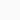# Measurement of Physical Quantity and Unit: NotesBy: Stupid
Updated:
Physics

What is Physical Quantity? What is Unit? A brief & conceptual study on physical quantities and their units for class 9 and 11 students. It is also useful for aspirant of UPSC prelims and other competitive government job exams.

## Physical Quantity

Physical Quantities are those which are measurable. Mass, length, time, speed, force, etc are some examples of physical quantities. Here, the mass measure the quantity of matter, time measures the duration. Similarly, speed measures that how fast something moves.

There are two parts in a physical quantity, one is ‘number‘ and another is ‘unit‘. The number indicates “how much” the quantity is there. The unit helps us to identify the “which physical quantity is this“.

## Unit:

If you go to a grocery store and ask the shopkeeper to give potato, then the shopkeeper will definitely ask you a question – “How much?” If your answer is something like 5, then again “what 5?” So, basically just saying a number does not make any sense. To make it sensible or meaningful we need to put something after the number 5. So what we put after the number is called “unit“. Therefore you need to specify the unit (kilogram i.e. 5 Kg) so that people can understand the exact amount of physical quantity.

The unit helps us to know the exact amount of physical quantity.
The unit of a given physical quantity is unique.

The unit represents a standard quantity of that physical quantity. As an example, the length of a rod = 5 m (meter). This means 5 multiplied by 1 meter. So here length is a physical quantity, its numerical value is 5 and unit is m (meter). Here 1 m refers to a certain amount of predefined length. 5 m is 5 times this standard amount (1 m).

There are two types of units - 1. Fundamental unit 2. Derived unit.

Fundamental Unit: The units which are independent of other units are known as fundamental units.

Length, mass, and time – these are three base quantity and their units are base units or fundamental units (meter kilogram, second respectively).

Derived Unit: Derived units are derived from fundamental units.

Velocity, force, momentum, density, etc are derived quantity and their units are derived units (m/s N, Kg-m/s, Kg/cubic meter respectively).

## System of Unit:

There are three methods used around the world for a long time, these are –

1. c.g.s = centemeter-gram-second
2. M.K.S = meter-kilogram-second
3. F.P.S = foot-pound-second

## Si Unit (International system)

In order to measure the same physical quantity in a separate single method, a separate measuring instrument has to be made for each method. Apart from that, it is not possible to disclose the data obtained from various measurements in a consistent manner. So to remove this problem the International Bureau of Weights and Measures adopted a single approach internationally to address this problem. This is known as the SI system (Système international).

## Symbol of Units:

Symbols are used to abbreviate each unit. A tradition of how to write different symbols has been recognized internationally. There are some rules to write units.

1. The dot marks can not be placed between or after the symbol. Example: centemeters: cm (not c.m)
2. S or es cannot be added to denote plural. E.g 5 gram = 5 g (not 5 gs).
3. The first letter of the unit symbol found in the names of the scientists will be capitalized, the other symbol will use only lower case. Example: force = 5 Newtons = 5 N. [ kelvin- K, Pascal -Pa, Celsius-° C].
4. Units should follow the division and multiplication rules of algebra (cm ✖ cm = cm2).
5. The degree symbol (°) should not be used to express the unit of temparature in kalvin unit (should use only K).

## List of 7 Base Quantities and Units:

The term “unit less quantity” refers to a condition in which no fundamental quantities are involved. It doesn’t have a unit, hence it doesn’t have any dimensions.

## Supplementary Units:

Supplementary units are dimensionless units. Angle (measured in radians) and solid angle (measured in steradian) are two supplementary units.